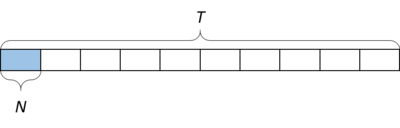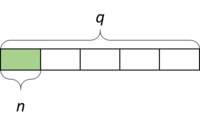# Reciprocity

Alignments to Content Standards: 6.NS.A 6.EE.A

Telula has 10 times as much iced tea as Nikki. We can represent this with a diagram:or a multiplication equation:

$$T=N \times 10$$

We can also see in the diagram that that Nikki has $\frac{1}{10}$ times as much iced tea as Telula. We can represent this with a multiplication equation as well:

$$N = T \times \frac{1}{10}$$

Consider the following three pairs of sentences for the questions below:

• A nickel is worth $\frac15$ times as much as a quarter; $n=q \times \frac15$.
A quarter is worth _____ as much as a nickel; _________________.
• Hilo's pig weighs $\frac23$ times as much as Hilo's dog; $P=D \times \frac23$.
Hilo's dog weighs _____ as much as Hilo's pig; _________________.
• Henry ate $\frac53$ times as much ice cream as Cecil; $H=C \times \frac53$.
Cecil ate _____ as much ice cream as Henry; _________________.

1. For the first sentence of each pair, draw a picture that represents the sentence and equation.
2. For the second sentence of each pair, fill in the blank and write a multiplication equation.

In the original problem above, we could write $T=N \times 10$ as $N = T\div 10$.

1. Rewrite each of the original equations as a division equation in the second column.
2. Rewrite your equation in the third column using multiplication (look at the sample row).
 Original Equation Dividing Multiplying $T=N \times 10$ $N = T\div 10$ $N = T\times \frac{1}{10}$ $n=q \times \frac15$ $P=D \times \frac23$ $H=C \times \frac53$
1. Describe a pattern you can see with the Dividing and Multiplying equations.

## IM Commentary

The purpose of this task is to help students understand why dividing by a fraction gives the same result as multiplying by its reciprocal. This is accomplished by writing the division equation along with related multiplication equations and diagrams showing the situation for several different contexts. Then, the related equations are organized in a table so that students can express regularity in repeated reasoning (MP8) to make the general observation that dividing by a fraction is the same as multiplying by its reciprocal.

This task builds on work in 5.NF.B where students draw diagrams and write equations to make sense of many contexts involving multiplication and division, so that drawing a diagram of $\frac53$ times as much as a quantity should not pose a huge hurdle to students engaging in this task (that skill isn't the focus of this task). To alleviate the need to draw a bunch of rectangles, though, the teacher might decide to provide students with a blank template to draw on.

Students may be inclined to write the products with the scale factor first. The products in the equations in the top (sample) row of the table are written in a particular order so that students can easily observe the intended pattern without having to mentally rearrange the products. If students write them in the other order, they can be reminded that multiplication is commutative and they might have an easier time answering the questions if they write them as they are written in the sample row. Alternatively, an exemplar table could be shown on a projector or written on a board for everyone to see as a class discussion takes place.

It is possible that in the third column some students may write, for example, $q=n \times \frac{1}{1/5}$ and $D=P \times \frac{1}{3/2}$. A teacher might use this opportunity to help students reconcile these equivalent forms. The diagrams produced in Part 1, combined with students' existing understanding from 5.NF.B, could be used to show why, for example $$\frac{1}{1/5}=1 \div \frac15=1 \times 5=5$$.

Work that follows this task might include solving problems using both a diagram and the "invert and multiply" algorithm and noting that they produce the same result. For example, see these tasks published in 6.NS.A.1

Eventually, of course, students should approach dividing by a fraction by applying the "invert and multiply" algorithm automatically and correctly without needing to draw a diagram.

## Solution

a. For the first sentence, draw a picture.
b. For the second sentence, fill in the blank and write a multiplication equation.

• A quarter is worth 5 times as much as a nickel.$$q = n \times 5$$
• Hilo's dog weighs $\frac32$ times as much as Hilo's pig.$$D = P \times \frac32$$
• Cecil ate $\frac35$ times as much ice cream as Henry.$$C = H \times \frac35$$

 Original Equation Dividing (Answers to c.) Multiplying (Answers to d.) $T=N \times 10$ $N = T\div 10$ $N = T\times \frac{1}{10}$ $n=q \times \frac15$ $q = n\div \frac15$ $q = n\times 5$ $P=D \times \frac23$ $D = P\div \frac23$ $D = P\times \frac32$ $H=C \times \frac53$ $C = H\div \frac53$ $C = H\times \frac35$

e. If we put the second two equations together, we can see a pattern:

$T\div 10 = T \times \frac{1}{10}$

$n \div \frac15 = n \times 5$

$P \div \frac23 = P \times \frac32$

$C \div \frac53 = C \times \frac35$

When we divide by a number, we get the same answer as when we multiply by its reciprocal.